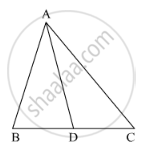Share

In the Following Figure, Check Whether Ad is the Bisector of ∠A of ∆Abc in the Following Case: Ab = 5 Cm, Ac = 12 Cm, Bd = 2.5 Cm and Bc = 9 Cm - CBSE Class 10 - Mathematics

Question

In the following figure, check whether AD is the bisector of ∠A of ∆ABC in the following case:

AB =  5 cm, AC = 12 cm, BD = 2.5 cm and BC = 9 cmSolution

It is given that AB = 5 cm, AC = 12 cm, BD = 2.5 cm and BC = 9 cm

We have to check whether AD is bisector of ∠A.

First we will check proportional ratio between sides.

Now

"AB"/"AC"=5/12

"BD"/"CD"=2.5/9=5/18

Since "AB"/"AC"!="BD"/"CD"

Hence AD is not the bisector of ∠A.

Is there an error in this question or solution?

Video TutorialsVIEW ALL 

Solution In the Following Figure, Check Whether Ad is the Bisector of ∠A of ∆Abc in the Following Case: Ab = 5 Cm, Ac = 12 Cm, Bd = 2.5 Cm and Bc = 9 Cm Concept: Angle Bisector.
S